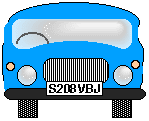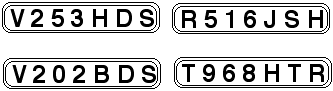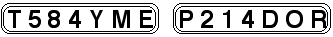#### You may also like### Prompt Cards

These two group activities use mathematical reasoning - one is numerical, one geometric.### Consecutive Numbers

An investigation involving adding and subtracting sets of consecutive numbers. Lots to find out, lots to explore.### Exploring Wild & Wonderful Number Patterns

EWWNP means Exploring Wild and Wonderful Number Patterns Created by Yourself! Investigate what happens if we create number patterns using some simple rules.

# Numbered Cars

## Numbered Cars

I was looking at the number plate of a car parked outside my house - S208VBJ.Using my special code S208VBJ adds to $65$.They all also add to $65$ using my code!

Using the code you treat every character, that is a digit or a letter, as separate.

Numbers are simply added together so that, in the first number plate $208$ is $2 + 0 + 8 = 10$.

Letters are translated into numbers in an organised and systematic way. That means that if you know the value of F and H you not only know the value of G, but can easily work out all the rest of the alphabet.

Can you crack my code and use it to find out what both of these number plates add up to?### Why do this problem?

This problem practises working systematically on addition and subtraction. This is a code, not algebra, and learners will need to try things out as well as working them out, so using trial and improvement will be essential.

### Key questions

Have you remembered that this is a code?
Have you thought of looking at the two number plates beginning with V?
Have you thought of writing out the alphabet and thinking of a way to turn the letters into numbers?
Can you think of a variation on the most obvious way of turning letters into numbers?

### Possible extension

Learners who found this problem easy could try Ishango Bone.

### Possible support

Suggest starting by writing out the numbers on the number-plates and adding up each one. Then write out the alphabet and think of a way of varying A = $1$, B = $2$ etc.